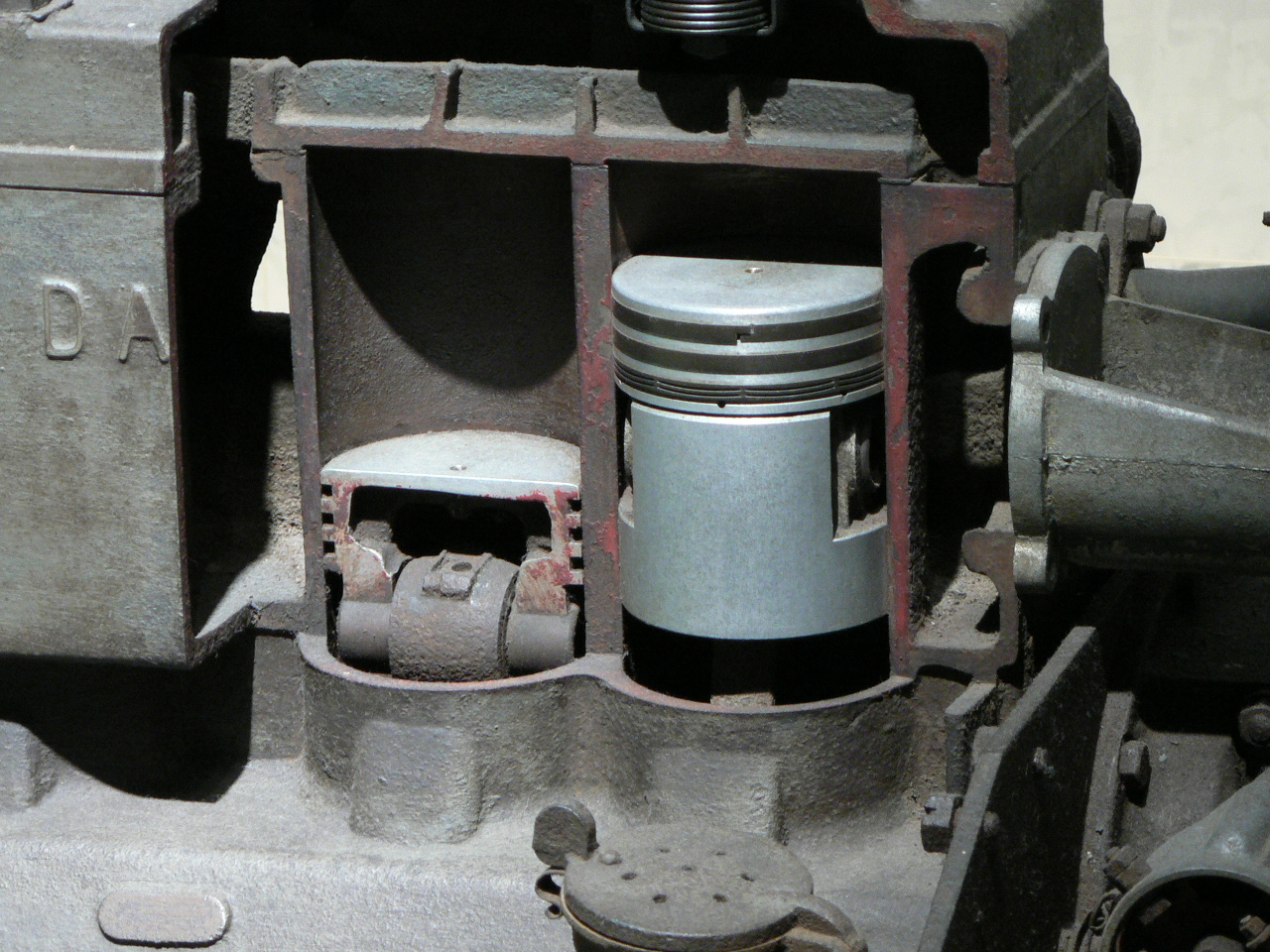Lecture slides     |     Basic equations     |      Text     |     Tools     |     Help

#### Heat and WorkConservation of energy## The first law of thermodynamics is a law of conservation of energy. It tells us heat and work can be interconverted. There are several kinds of work we could consider, but the most important one for this course will be pressure-volume work. There is also electrical, gravitational and even magnetic work. But, these are considered in advanced applications. Pressure-volume work is the kind of work done in an internal combustion engine, for example. When fuel such as octane is combusted in a dylinder it cases a piston to move and thereby changes the volume. The same principle applies to a steam engine and other types of engine. We will consider how to take the definition of work that you may know from basic physics, i.e. work is a force operating through a distance and convert that to a pressure operating to change a volume. These definitions are interchangable. An engine is driven by heat, which comes from combustion so the principle of operation of an engine is an example of the first law of thermodynamics.In the topics on the right we begin with the definition of the internal energy of an ideal gas as the starting point. An ideal gas has no potential energy since the assumption is that there is no interaction between particles. This means that all of the energy is kinetic energy as we saw in the previous lecture. Based on the idea that the kinetic energy depends on the temperature we can write U = 3/2 nRT for an ideal gas, where U is the internal energy. The change in the internal energy of an ideal gas can arise from a change in temperature (adding or removing heat) and doing work (compressing or expanding to change the motion of the gas). We can write Delta U = q + w, where q is the heat transferred and w is the work done. Using this definition we will consider the thermodynamic sign convention, which states that the sign of a change will be negative if the energy of the system decreaes and positive if the energy of the system increases. Finally, we can show how to graphically represent work done on a system or by a system.Reversible and irreversible work XXX XXX

problem solving### Practice as you go...### States and Paths